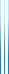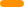# PublicationsPapers

## The complexity of the comparator circuit value problem

Stephen A. Cook, Yuval Filmus and Dai Lê
TOCT

In 1990 Subramanian defined the complexity class $\mathsf{CC}$ as the set of problems log-space reducible to the comparator circuit value problem (CCV). He and Mayr showed that $\mathsf{NL}\subseteq \mathsf{CC}\subseteq \mathsf{P}$, and proved that in addition to CCV several other problems are complete for $\mathsf{CC}$, including the stable marriage problem, and finding the lexicographically first maximal matching in a bipartite graph. Although the class has not received much attention since then, we are interested in $\mathsf{CC}$ because we conjecture that it is incomparable with the parallel class $\mathsf{NC}$ which also satisfies $\mathsf{NL}\subseteq \mathsf{NC}\subseteq \mathsf{P}$; this implies that $\mathsf{CC}$-complete problems don’t have an efficient polylog time parallel algorithm. We provide evidence for our conjecture by giving oracle settings in which relativized $\mathsf{CC}$ and relativized $\mathsf{NC}$ are incomparable.

We give several alternative definitions of $\mathsf{CC}$, including (among others) the class of problems computed by uniform polynomial-size families of comparator circuits supplied with copies of the input and its negation, the class of problems $\mathsf{AC^0}$-reducible to CCV, and the class of problems computed by uniform $\mathsf{AC^0}$ circuits with CCV gates. We also give a machine model for $\mathsf{CC}$ which corresponds to its characterizations as log-space uniform polynomial-size families of comparator circuits. The various characterizations show that $\mathsf{CC}$ is a robust class. Our techniques also show that the corresponding function class FCC is closed under composition. The main technical tool we employ is universal comparator circuits.

Other results include a simpler proof of $\mathsf{NL}\subseteq \mathsf{CC}$, a more careful analysis showing that the lexicographically first maximal matching problem and its variants are $\mathsf{CC}$-complete under $\mathsf{AC^0}$ many-one reductions, and an explanation of the relation between the Gale–Shapley algorithm and Subramanian’s algorithm for stable marriage.

This paper continues previous work of Cook, Lê and Ye which focused on Cook–Nguyen style uniform proof complexity, answering several open questions raised in that paper.

The preprint contains more results than the arXiv version, and the presentation is different.

## BibTeX

@article{CFL2014,
author = {Stephen A. Cook and Yuval Filmus and Dai L\^e},
title = {The complexity of the comparator circuit value problem},
journal = {ACM Transactions on Computation Theory},
volume = {6},
number = {4},
articleno = {15},
pages = {15:1--15:44},
year = {2014}
}copy to clipboard